#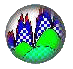Interactive Real Analysis - part of MathCS.org

Next | Previous | Glossary | Map | Discussion

## Example 4.1.10: The Leaning Tower of Lire

Jillian, a diligent but overworked student, fell asleep in the library and got locked in for the night. When she awoke, the room was dimly lit and she was alone. To pass the time (and to annoy the librarian in the morning) she decided to stack books on a table so that they would overhang the edge of the table.

Assuming she has an unlimited supply of books, all of equal width 2 and weight 1 (say), what is the biggest overhang she can produce? To make it more interesting, let's say she can use only one book at each level.

This problem is sometimes known as the "Leaning Tower of Lire" problem and has a number of available solutions (see references at the end).

Jillian quickly has the idea of simply stacking books vertically near the edge of the table. After stacking a few books she pushes the entire stack so that part of the books overhang the edge of the table.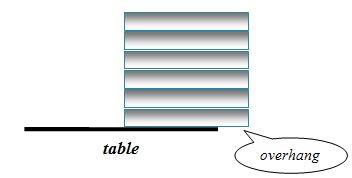This will work, follows the rules, and (since the books have width 2) it is easy to see that while the stack can be arbitrarily high, the largest overhead that can be achieved this way is just about 1. Not much - the librarian in the morning would be annoyed but not impressed.

Jillian thinks again and comes up with a more imaginative way of stacking the books: she uses 'counter-weights' to produce a large overhang, as shown in the picture below.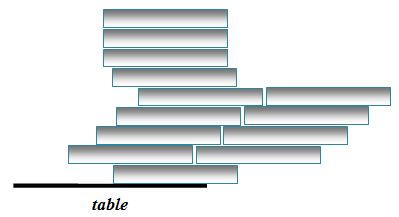This can produce a larger overhang indeed, but unfortunately uses more than one book per level. Thus, this idea is against the rules and has to be discarded.

Now Jillian remembers her math background and attacks the problem analytically. Recall that the combined center of gravity c of two objects with (point) mass M1 and M2, located at x1 and x1, respectively, is: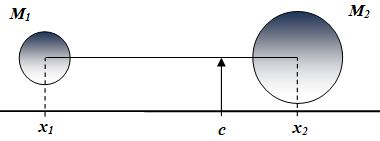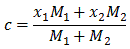To model our problem, let's imagine a number line extending to the right of the table so that the origin is at the right edge of the table.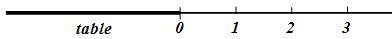We can assume that a stack of n-blocks will not fall off the table as long as its center of gravity cn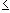0. In particular, the center can be at zero and the stack will still (barely) balance on the edge. Now let's start with an empty table. We will stack our blocks as follows:

### Step 1

Put block 1 on the table so that its right edge is at zero. The block has width 2, mass 1, and center of gravity -1.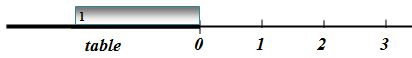Now we will shift the block until the center of gravity is at zero so that it still barely stays on the table. Since the block has width 2 and center of gravity -1 we can shift it half its width (1 unit) to the right and it will still remain on the table.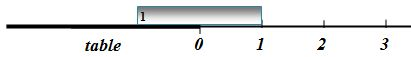Stack 1:

• Mass: M1 = 1
• Center of gravity: c1 = 0
• Overhang: D1 = 1

### Step 2

Lift the existing stack (one book) straight up. Put a new block on the table as before, so that its right edge is at zero. Put down the existing stack to get a new stack of 2 blocks. It will remain balanced on the new block.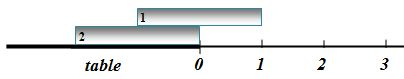The center of gravity of this size-2 stack is: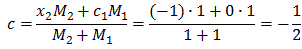Now shift the block right until its center of gravity is at zero. Since the stack's center of gravity is currently at -1/2 we can shift the stack right by that amount.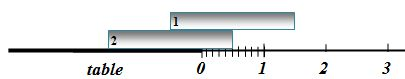Stack 2:

• Mass: M2 = 2
• Center of gravity: c2 = 0
• Overhang: D2 = 1+1/2

### Step 3

Again lift the existing stack straight up. Put a new block on the table as before, so that its right edge is at zero. Put down the existing stack to get a new stack of 3 blocks. It will remain balanced on the new block.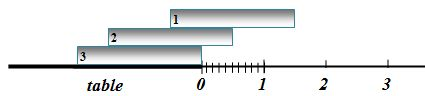The center of gravity of this size-3 stack is: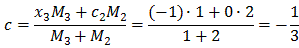Then shift the stack until the center of gravity is at zero. Since the stack's center of gravity is -1/3 we can shift the stack right by that amount.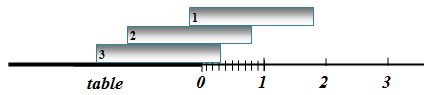Stack 3:

• Mass: M3 = 3
• Center of gravity: c3 = 0
• Overhang: D3 = 1+1/2+1/3

### Step 4

Let's do it one more time: lift the existing stack straight up and put a new block on the table so that its right edge is at zero. Drop down the existing stack to get a new stack of 4 blocks. It will remain balanced on the new block.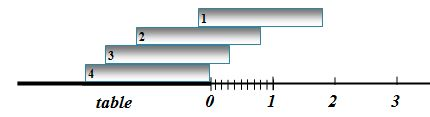The center of gravity of this size-4 stack is:Then shift the stack until its new center of gravity is at zero. Since the stack's center of gravity right now is -1/4 we can shift the stack right by that amount.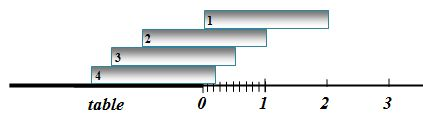Stack 4:

• Mass: M4 = 4
• Center of gravity: c4 = 0
• Overhang: D4 = 1+1/2+ 1/3+ 1/4

### Step n

Now we have the algorithm down: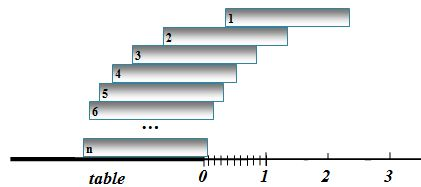• Lift up the existing stack Sn with n blocks, center of gravity 0, and overhang Dn = 1+1/2+...+1/n
• Put down a new block bn+1 with right edge at zero
• Drop down the stack to form a new stack Sn+1 with n+1 blocks
• Compute the center of gravity of the new stack Sn+1: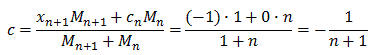• Shift stack Sn+1 to the right so that its new center of gravity will be zero. The overhang of stack Sn+1 will then be:
Dn+1 = 1 + +1/2 + ... + +1/n+1

Thus, we created recursively a stack of N blocks that (just barely) balances on the table yet will overhang the edge of the table by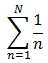. Since this is the N-th partial sum of the divergent harmonic series, we can create an overhang as large as we wish - we might need a lot of blocks, though. For example:

 Desired overhang Blocks needed (N) 2 N = 4 4 N = 31 10 N = 12,367 22 N = 2,012,783,315 40 N = 132,159,290,357,566,703

This is a theoretical result but if you have actual blocks of equal size (made of wood, for example) and you work through this algorithm very carefully, you can achieve truely astonishing overhangs - perhaps not 22 or more but certainly enough impress any librarian!

This version of the "Leaning Tour of Lires" has been created by with the help of Jillian Gaglione, a former Seton Hall student, as part of an independent studies project. And no, she did not really fall asleep in the libray - so she says.

### References

Next | Previous | Glossary | Map | Discussion